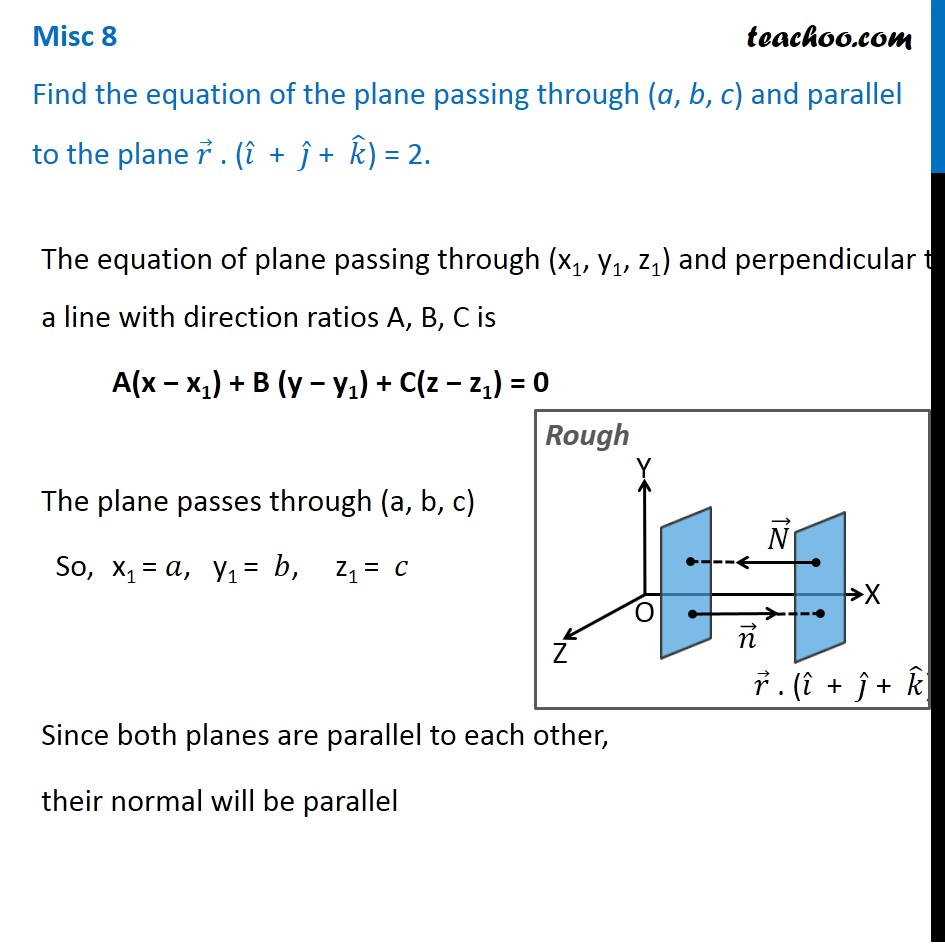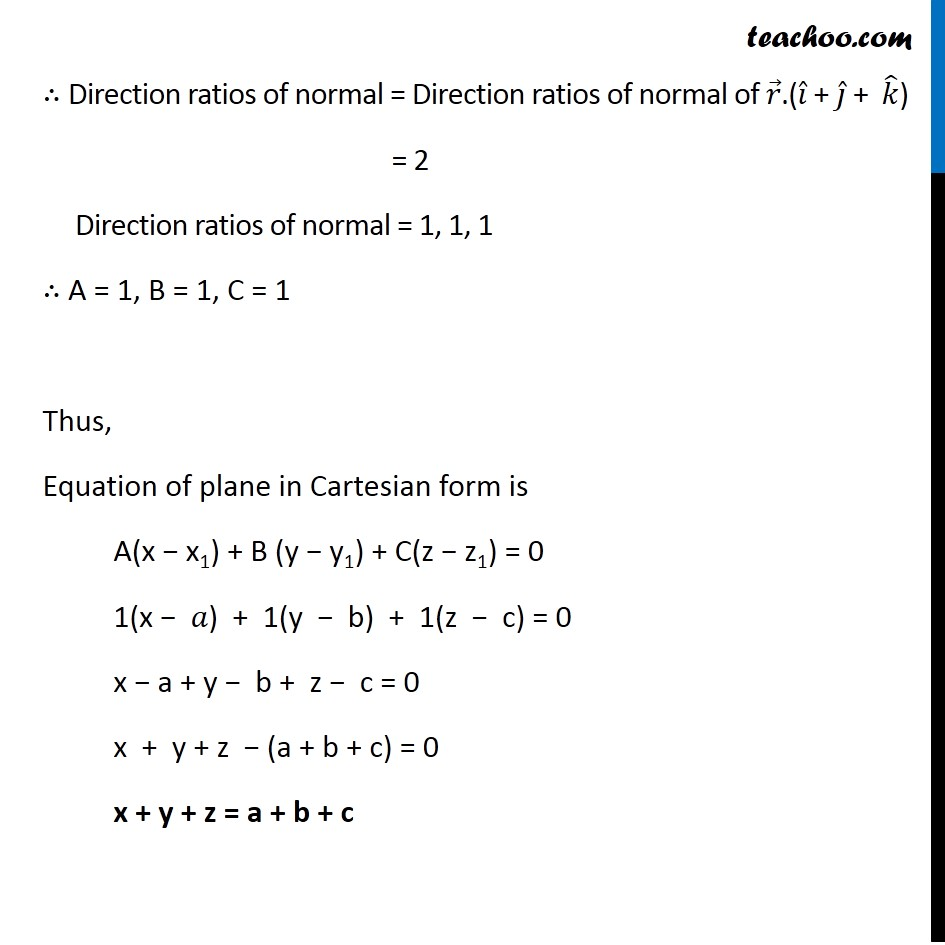Miscellaneous

Chapter 11 Class 12 Three Dimensional Geometry
Serial order wiseLearn in your speed, with individual attention - Teachoo Maths 1-on-1 Class

### Transcript

Question 5 Find the equation of the plane passing through (a, b, c) and parallel to the plane 𝑟 ⃗ . (𝑖 ̂ + 𝑗 ̂ + 𝑘 ̂) = 2.The equation of plane passing through (x1, y1, z1) and perpendicular to a line with direction ratios A, B, C is A(x − x1) + B (y − y1) + C(z − z1) = 0 The plane passes through (a, b, c) So, x1 = 𝑎, y1 = 𝑏, z1 = 𝑐 Since both planes are parallel to each other, their normal will be parallel ∴ Direction ratios of normal = Direction ratios of normal of 𝑟 ⃗.(𝑖 ̂ + 𝑗 ̂ + 𝑘 ̂) = 2 Direction ratios of normal = 1, 1, 1 ∴ A = 1, B = 1, C = 1 Thus, Equation of plane in Cartesian form is A(x − x1) + B (y − y1) + C(z − z1) = 0 1(x − 𝑎) + 1(y − b) + 1(z − c) = 0 x − a + y − b + z − c = 0 x + y + z − (a + b + c) = 0 x + y + z = a + b + c ∴ Direction ratios of normal = Direction ratios of normal of 𝑟 ⃗.(𝑖 ̂ + 𝑗 ̂ + 𝑘 ̂) = 2 Direction ratios of normal = 1, 1, 1 ∴ A = 1, B = 1, C = 1 Thus, Equation of plane in Cartesian form is A(x − x1) + B (y − y1) + C(z − z1) = 0 1(x − 𝑎) + 1(y − b) + 1(z − c) = 0 x − a + y − b + z − c = 0 x + y + z − (a + b + c) = 0 x + y + z = a + b + c